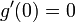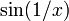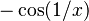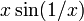# Derivative of differentiable function need not be continuous

This article gives the statement and possibly, proof, of a non-implication relation between two function properties. That is, it states that every function satisfying the first function property (i.e., differentiable function) need not satisfy the second function property (i.e., continuously differentiable function)
View a complete list of function property non-implications | View a complete list of function property implications
Get more facts about differentiable function|Get more facts about continuously differentiable function
EXPLORE EXAMPLES YOURSELF: View examples of subgroups satisfying property differentiable function but not continuously differentiable function|View examples of subgroups satisfying property differentiable function and continuously differentiable function

## Statement

It is possible to have a function$f$ defined for real numbers such that$f$ is a differentiable function everywhere on its domain but the derivative$f'$ is not a continuous function.

Equivalently, a differentiable function on the real numbers need not be a continuously differentiable function.

## Related facts

• Difference quotient: We can relate the continuity of the derivative to the joint continuity of the difference quotient.

## Proof

### Example with an isolated discontinuity

Consider the function:$g(x) := \left\lbrace\begin{array}{rl} x^2 \sin(1/x), & x \ne 0 \\ 0, & x = 0 \\\end{array}\right.$

Then, we have:$g'(x) = \left\lbrace\begin{array}{rl} 2x \sin(1/x) - \cos(1/x) & x \ne 0 \\ 0, & x = 0 \\\end{array}\right.$

In particular, we note that$g'(0) = 0$ but$\lim_{x \to 0} g'(x)$ does not exist. Thus,$g'$ is not a continuous function at 0.

For details, see square times sine of reciprocal function#First derivative.

#### Thinking to motivate the example

The basic idea for the example comes from the observation that derivative of differentiable function satisfies intermediate value property. This means that for any counterexample, the derivative would still satisfy the intermediate value property. The next step is to therefore look for examples showing that intermediate value property not implies continuous. We see that the example used there is$\sin(1/x)$.

Unfortunately we don't have an elementary expression for the antiderivative of the sine of reciprocal function. However, we don't quite need to get an antiderivative of that function -- we just need something whose derivative is a sum of something that clearly goes to zero, and something of the structure of$\sin(1/x)$ (for instance,$-\cos(1/x)$ will also do).

The first attempt might be$x \sin (1/x)$, since integration gets you one degree higher. However, for this function, the derivative at zero doesn't exist -- the challenge comes from the$-1/x^2$ appearing when we apply chain rule to differentiate$\sin(1/x)$. We therefore need a higher degree to cancel that$-1/x^2$. We next try$x^2 \sin(1/x)$, and that works.